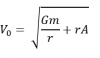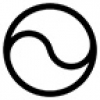## Hypersphere CosmologyAlternative to big-bang

Thursday, 04 December 2014 09:15

## Hypersphere RotationThe Gödel 3d rotating universe has this solution derived from General Relativity: -“Matter everywhere rotates relative to the compass of inertia with an angular velocity equal to twice the square root of pi times the gravitational constant times the density.” – Gödel.

So, squaring and substituting mass over volume for d yields:= 4πGm/v

Substituting 4/3 πfor the volume of a sphere yields:Substitutingthe formula for a photon sphere (where orbital velocity equals lightspeed) yields:Taking the square root:

(1*)  W =Substituting L for r as we wish to find the solution for a hypersphere:

(2*) W =Squaring yields:Substituting GM/L =the formula for a hypersphere yields:

W=Which we can also express as:

W=or as W =Substituting W = 2πf  in (1*) and (2*), to turn radians into frequency we obtain:for a sphere and f =for a hypersphere.

Centripetal acceleration a, where a =for a sphere and afor a hypersphere, yields:= for a sphere and a == for a hypersphere.

Now for a hypersphere wherea centrifugal acceleration a, of a =or more simply a =will exist, to exactly balance the centrifugal effects of rotation.

In a hypersphere of about L = 13.8 billion light years, the rotation will correspond to about 0.0056 arcseconds per century. As this consists of a 4-rotation of the 3d ‘surface’, visualisation or measurement of its effects may prove problematical, the rotation should eventually cause every point within the 3d ‘surface’ to exchange its position with its antipode point, over a 13.8 billion year period, turning the universe into a mirror image of itself.

Note that if the universe does have the same number of dimensions of time as of space then it will rotate back and forth between matter and anti-matter as well.

A 4-rotation does not submit to easy visualisation but consider this: -

A needle rotated in front of an observer and viewed in the plane of rotation will appear to shrink to a small point and then become restored to its original length with further rotation.

Likewise a sheet of card will appear to shrink to a line and then expand back into a plane if rotated in front of an observer.

In both cases the apparent shrinkage and expansion depends on the observer not seeing the needle or card in their full dimensionality.

A cube (or a sphere) rotated through a fourth dimension would appear to shrink to a point and then expand back to its original size to an observer viewing it in only three dimensions and ignoring the curvature.

• #### HyperSphere Cosmology +Hypersphere Cosmology in a Nutshell   1a) The Universe consists of a Hypersphere (a 3-Sphere or 4-ball) where: -       also
• #### Hypersphere Redshift +The alternative mechanism for redshift works as follows: Redshift = Z =   - 1 Where = observed wavelength, = expected wavelength, and the -1
• #### Hypersphere Rotation +The Gödel 3d rotating universe has this solution derived from General Relativity: - “Matter everywhere rotates relative to the compass of
• #### Hypersphere Holometry - A Prediction +As a consequence of the hypothesis that the universe exists as a hypersphere where   the main parameters of the
• #### VHC. Vorticitating Hypersphere Cosmology +The overwhelming majority of university and state funded cosmologists believe that the observable universe has expanded from a much smaller
• #### Hypersphere Visualisation & Lensing +This paper provides a method of visualising the four dimensional hypersphere as a perspective construction in three dimensions, and it
• #### Sacred Geometry +

As faith began to make way for reason during the Enlightenment, humans began to deduce the relationships between certain mathematical
• #### Starships +

Houston we have a problem. If the human race does not develop starships that can reach other star systems as
• #### Quantum & Probability +

I have repeatedly asserted in many of my magical and scientific books and papers that this universe runs on probability
• #### Ur Chaos Theory +

In this paper 'Chaos' denotes randomness and indeterminacy, not the mere so-called 'deterministic chaos' of systems with extreme sensitivity to
• 1